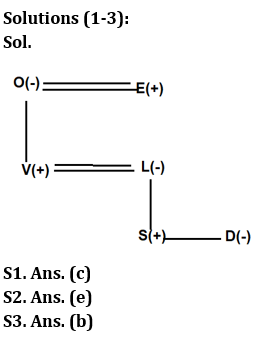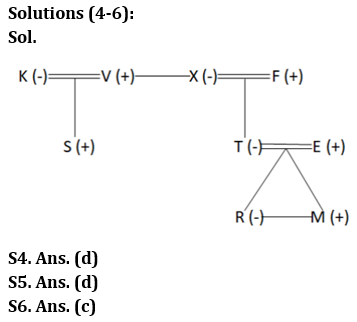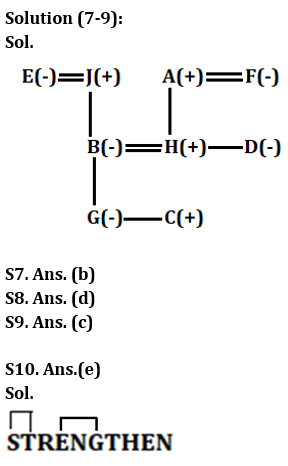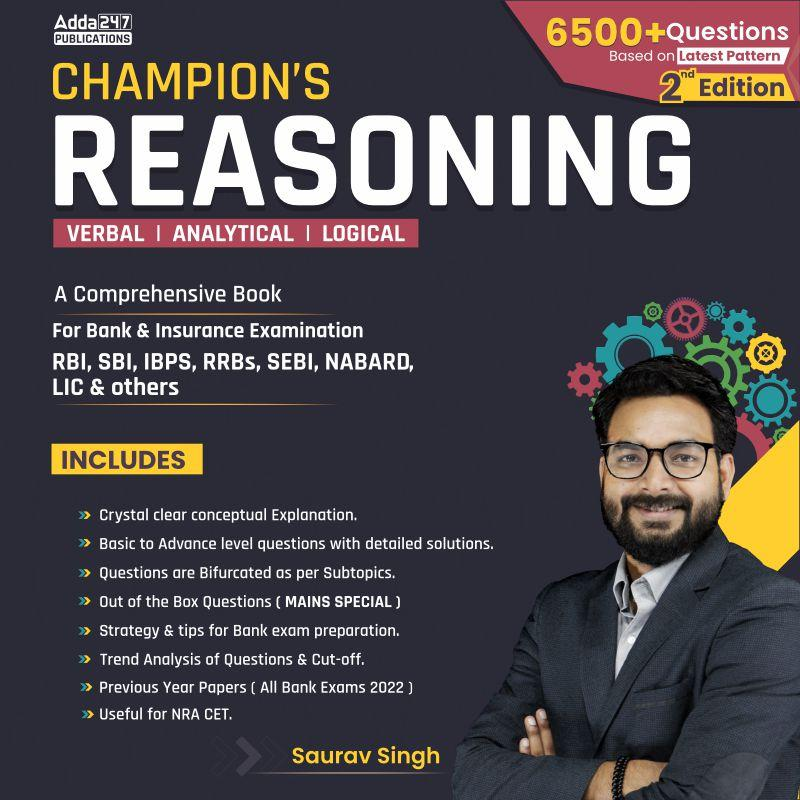Latest Banking jobs   »   IBPS reasoning

# Reasoning Quiz For IBPS PO Prelims 2023-19th September

Directions (1-3): Study the information carefully and answer the questions accordingly.
S, O, L, V, E and D are six members in a family of three generations. There are two married couples among them. L is the mother of S and D. E is the father of V. S is the grandson of O. The total number of female members in the family is three. V is a married person with two children.

Q1. Which of the following pair is one of the married couples?
(a) E-D
(b) O-V
(c) E-O
(d) S-D
(e) None of these

Q2. How is D related to S?
(a) Brother
(b) Daughter
(c) Son
(d) Mother
(e) None of these

Q3. Which of the following is a group of male members?
(a) OVE
(b) SVE
(c) VLD
(d) OLD
(e) None of these

Directions (4-6): Study the information carefully and answer the questions accordingly.
T is the only child of F. F has no sister. E is the son-in-law of X. V is the brother-in-law of F. X does not have a niece. K and V is a couple. S is the cousin of T. R is the granddaughter of X. M is the son of T. X is the wife of F.

Q4. How V is related to T?
(a) Niece
(b) Nephew
(c) Father
(d) Uncle
(E) None of these

Q5. How many female members are there?
(a) Three
(b) One
(c) Two
(d) Four
(e) Can’t be determined

Q6. How F is related to M?
(a) Nephew
(b) Uncle
(c) Grandfather
(d) Father
(e) None of these

Directions (7-9): Study the information carefully and answer the questions accordingly.
A three-generation family consists of nine members i.e. A, B, C, D, E, F, G, H and J where the number of females are more than the number of males. D is the sister of H. B is the mother of G. J is the grandfather of G. F is the mother of H. A is the father-in-law of B. C is the sibling of G. A’s spouse have only two children. C is the grandson of H’s mother-in-law.

Q7. What is the relation of F with respect to B?
(a) Maternal Aunt
(b) Mother-in-law
(c) Niece
(d) Daughter-in-law
(e) Mother

Q8. If N is the husband of G, then what is the relation of N with respect to H?
(a) Son
(b) Father-in-law
(c) Brother-in-law
(d) Son-in-law
(e) None of these

Q9. Which of the following is true with respect to the given arrangement?
(a) F is the mother of D
(b) D and H are sisters.
(c) Only five female members are there in the family.
(d) J has two granddaughters.
(e) All are true

Q10. How many such pairs of letters (both forward and backward direction) are there in the word “STRENGTHEN” each of which has as many letters between them in the word as they have between them in the English alphabetical series?
(a) One
(b) Three
(c) None
(d) More than three
(e) Two

Solutions## FAQs

### When will the IBPS PO prelims 2023 be conducted?

IBPS PO Prelims will be conducted on 23, 30 September, and 1 October 2023.

#### Congratulations!Union Budget 2023-24: Free PDF